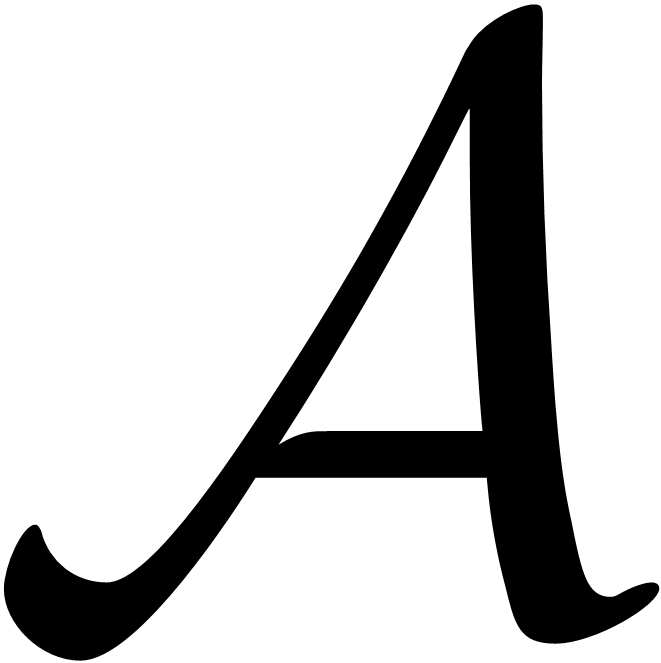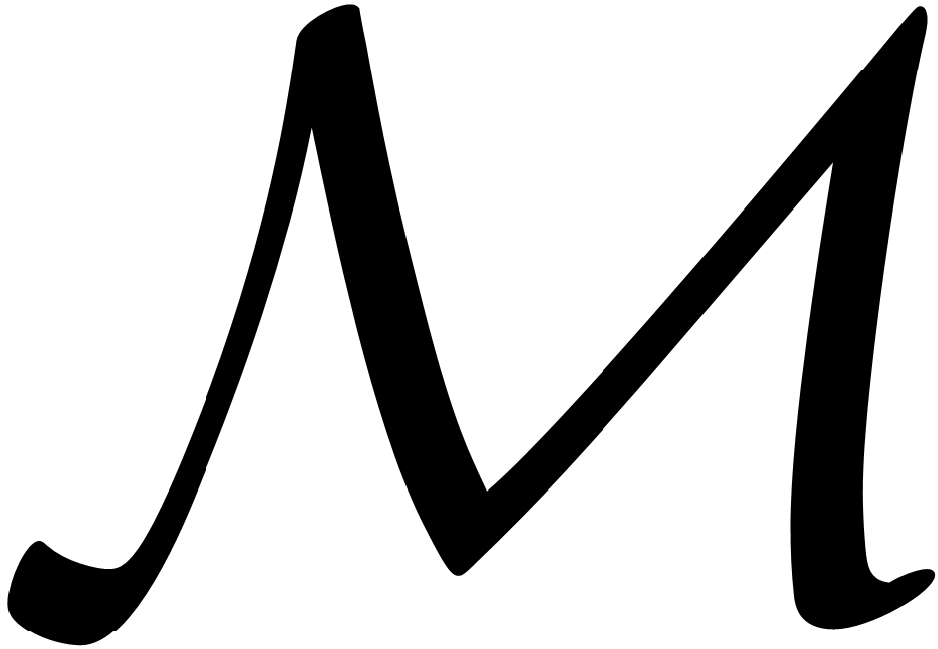Archive
Special IssuesVolume 9, Issue 5, October 2020, Page: 96-100
Characterizations of Jordan *-derivations on Banach *-algebras
Guangyu An, Department of Mathematics, Shaanxi University of Science and Technology, Xi’an, China
Ying Yao, Department of Mathematics, Shaanxi University of Science and Technology, Xi’an, China
Received: Aug. 11, 2020;       Accepted: Sep. 18, 2020;       Published: Oct. 28, 2020
Abstract
Suppose thatis a real or complex unital Banach *-algebra,is a unital Banach-bimodule, and G ∈is a left separating point of. In this paper, we investigate whether the additive mapping δ:satisfies the condition A,B, AB = G ⇒ Aδ(B)+δ(A)B*= δ(G) characterize Jordan *-derivations. Initially, we prove that ifis a real unital C*-algebra and G = I is the unit element in, then δ (non-necessarily continuous) is a Jordan *-derivation. In addition, we prove that ifis a real unital C*-algebra and δ is continuous, then δ is a Jordan *-derivation. Finally, we show that ifis a complex factor von Neumann algebra and δ is linear, then δ (non-necessarily continuous) is equal to zero.
Keywords
Jordan *-derivation, Left Separating Point, C*-algebra, Factor
Guangyu An, Ying Yao, Characterizations of Jordan *-derivations on Banach *-algebras, Pure and Applied Mathematics Journal. Vol. 9, No. 5, 2020, pp. 96-100. doi: 10.11648/j.pamj.20200905.13
Reference

M. Breˇ sar and J. Vukman. On some additive mappings in rings with involution, Aequ. Math., 3 (1989), 178-185.

M. Breˇ sar and B. Zalar. On the structure of Jordan ∗-derivations, Colloq. Math., 63 (1992), 163-171.

Q. Chen, C. Li, X. Fang. Jordan (α,β)-derivations on CSL subalgebras of von Neumann algebras, Acta. Math. Sin., 60 (2017), 537-546.

S. Goldstein and A. Paszkiewicz, Linear combinations of projections in von Neumann algebras, Proc. Amer. Math. Soc., 116 (1992), 175-183.

J. He, J. Li, D. Zhao, Derivations, Local and 2-local derivations on some algebras of operators on Hilbert Csup>*-modules, Mediterr. J. Math., 14, 230 (2017).

S. Kurepa. Quadratic and sesquilinear functionals, Glasnik Mat. Fiz.-Astronom, 20 (1965), 79-92. 106 Guangyu An and Ying Yao: Characterizations of Jordan ∗-derivations on Banach ∗-algebras

L. Liu. 2-Local Lie derivations of nest subalgebras of factors, Linear and Multilinear algebra, 67 (2019), 448-455.

D. Liu, J. Zhang. Local Lie derivations on certain operator algebras, Ann. Funct. Anal., 8 (2017), 270-280.

X. Qi and X. Zhang. Characterizations of Jordan ∗- derivations by local action on rings with involution, Journal of Hyperstructures, 6 (2017), 120-127.

P. ˇSemrl. On Jordan ∗-derivations and an application,Colloq. Math., 59 (1990), 241-251.

P.ˇSemrl. Jordan ∗-derivations of standard operator algebras, Proc. Amer. Math. Soc., 120 (1994), 515-518.

P.ˇSemrl. On quadratic functionals, Bull. Austral. Math. Soc., 37 (1988), 27-28.

P. ˇSemrl. Quadratic functionals and Jordan ∗-derivations, Studia Math., 97 (1991), 157-165.

P. Vrbová, Quadratic functionals and bilinear forms, Casopis Pest. Mat., 98 (1973), 159-161.

J. Vukman, Some functional equations in Banach algebras and an application, Proc. Amer. Math. Soc., 100 (1987), 133-136.